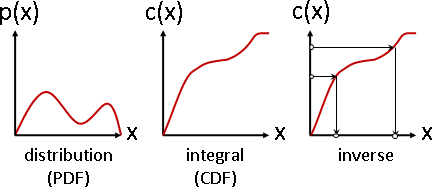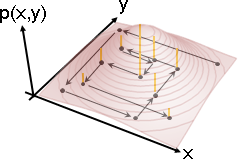Average Number

Weighted Average

```x = w x₁ + (1-w) x₂   (0 ≤ w ≤ 1)
```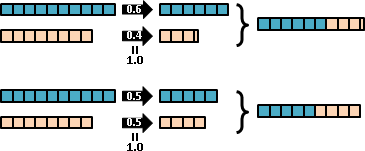```x = w₁x₁ + w₂x₂ + ... + wₙxₙ   (w₁ + ... + wₙ = 1)
```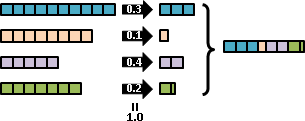```[x]      [x₁]      [x₂]            [xₙ]
[y] = w₁ [y₁] + w₂ [y₂] + ... + wₙ [yₙ]   (w₁ + ... + wₙ = 1)
[z]      [z₁]      [z₂]            [zₙ]

x = w₁ x₁ + w₂ x₂ + ... + wₙ xₙ
y = w₁ y₁ + w₂ y₂ + ... + wₙ yₙ
z = w₁ z₁ + w₂ z₂ + ... + wₙ zₙ
``````     (w₁)       (w₂)         (w₃)
a          b            c        ax₁ + bx₂ + cx₃
x = ————— x₁ + —————  x₂ +  ————— x₃ = ———————————————
a+b+c      a+b+c        a+b+c         a + b + c
```
```          a₁                       aₙ
x = ————————————— x₁ + ... + ————————————— xₙ
a₁ + ... + aₙ            a₁ + ... + aₙ

a₁ x₁ + ... + aₙ xₙ
= ———————————————————
a₁ + ... + aₙ
``````主角　　  加權平均數
--------  ----------

```

Floating Number

Random Variable與Distribution```     add: X+Y  (Cauchy product / convolution)
subtract: X-Y  (Cauchy product / convolution)
multiply: XY   (Dirichlet product)
divide: X/Y
``````一個隨機變數的

``````E[X+Y] = E[X] + E[Y]
E[X⋅Y] = E[X] ⋅ E[Y]   if X and Y are independent
E[X+k] = E[X] + k
E[X⋅k] = E[X] ⋅ k
Var[X+Y] = Var[X] + Var[Y] + Cov[X,Y]
Var[X-Y] = Var[X] + Var[Y] - Cov[X,Y]
Cov[X,Y] = 0   if X and Y are independent
Var[X+k] = Var[X]
Var[X⋅k] = Var[X] ⋅ k²
``````uniform distribution
Gauss distribution (normal distribution)
binomial distribution
Poisson distribution
```

Mixture Distribution（Mixture Model）Floating Tuple

Multivariate Random Variable與Joint Distributionl = {Line[{{3,3,1},{3,3,0}}], Line[{{5,6,1},{5,6,0}}], Line[{{8,2,1},{8,2,0}}], Line[{{2,8,1},{2,8,0}}]}; g = Show[ListPointPlot3D[{0,0,0}, Boxed -> False, Axes -> False, PlotRange -> {{0,10},{0,10}}], Graphics3D[{Thickness[0.01], CapForm["Butt"], Red, l}]] img = ImageResize[Rasterize[g, "Image", ImageResolution -> 72*3], Scaled[1/3]]; ImageCrop[RemoveBackground[img, {{{0, 0}}, 0.1}]]
F[x_, y_] := PDF[MultinormalDistribution[{3, 3}, {{1, 0}, {0, 1}}], {x, y}] + PDF[MultinormalDistribution[{5, 6}, {{1, 0}, {0, 1}}], {x, y}] + PDF[MultinormalDistribution[{8, 2}, {{1, 0}, {0, 1}}], {x, y}] + PDF[MultinormalDistribution[{2, 8}, {{1, 0}, {0, 1}}], {x, y}]; Show[ Plot3D[F[x,y], {x, 0, 10}, {y, 0, 10}, PlotRange -> All, Boxed -> False, Mesh -> None, Axes -> False, PlotPoints -> 50, ColorFunction -> (ColorData["CherryTones"][Rescale[#3, {-4, 4}]] &)], ParametricPlot3D[{x, 2, F[x,2]}, {x, 0, 10}, PlotStyle -> Red] ] Plot[F[x,2], {x, 0, 10}, PlotRange -> All, PlotStyle -> Red, Axes -> False] Show[ Plot3D[F[x,y], {x, 0, 10}, {y, 0, 10}, PlotRange -> All, Boxed -> False, Mesh -> None, Axes -> False, PlotPoints -> 50, ColorFunction -> (ColorData["CherryTones"][Rescale[#3, {-4, 4}]] &)], ParametricPlot3D[Table[{x, y, F[x,y]}, {y, 0.2, 9.8, 0.2}], {x, 0, 10}, PlotStyle -> Red] ] Plot[Integrate[F[x,y],{y,-20,+20}], {x, 0, 10}, PlotRange -> All, PlotStyle -> Red]

```條件分布 conditional distribution：部分維度只看特定數值。

```

Independent / UncorrelatedPlot3D[Piecewise[{{0.25, 1 < x < 3 && 1 < y < 3}}], {x, 0, 4}, {y, 0, 4}, PlotRange -> {0,1}, ExclusionsStyle -> Directive[Thick, Red], Boxed -> False, Mesh -> None, Axes -> False, PlotPoints -> 50, ColorFunction -> (ColorData["CherryTones"][Rescale[#3, {-4, 4}]] &)] F[x_,y_] := Piecewise[{ {0.15, 1 < x < 2 && 1 < y < 2}, {0.35, 2 < x < 3 && 1 < y < 2}, {0.35, 1 < x < 2 && 2 < y < 3}, {0.15, 2 < x < 3 && 2 < y < 3}}]; Plot3D[F[x,y], {x, 0, 4}, {y, 0, 4}, PlotRange -> {0,1}, ExclusionsStyle -> Directive[Thick, Red], Boxed -> False, Mesh -> None, Axes -> False, PlotPoints -> 50, ColorFunction -> (ColorData["CherryTones"][Rescale[#3, {-4, 4}]] &)]

```獨立　 independent ：p(X,Y)(x,y) = pX(x)pY(y)

```p = {{1, 1, 0.05}, {1.5, 1, 0.1}, {-1, -1, 0.1}, {-1, 0, 0.05}, {0, 1.5, 0.05}, {0, 0, 0.1}, {-1, -1.5, 0.2}, {1, -2, 0.15}, {-2, 1, 0.2}}; l = Table[Line[{p[[i]], {p[[i,1]], p[[i,2]], 0}}], {i,1,9}]; g = Show[ListPointPlot3D[{0,0,0}, Boxed -> False, Axes -> False, DataRange -> {{-2,2},{-2,2}}, PlotRange->{0,0.4}], Graphics3D[{Thickness[0.01], CapForm["Butt"], RGBColor[192,0,0], l}]] img = ImageResize[Rasterize[g, "Image", ImageResolution -> 72*3], Scaled[1/3]]; ImageCrop[RemoveBackground[img, {{{0, 0}}, 0.1}]]
px = {{2, 0.6}, {3, 0.25}, {2.5, 0.15}}; py = {{3, 0.6}, {2, 0.25}, {1 , 0.15}}; pxy = {{2, 3, 0.6*0.6}, {2, 2, 0.6*0.25}, {2, 1, 0.6*0.15}, {3, 3, 0.25*0.6}, {3, 2, 0.25*0.25}, {3, 1, 0.25*0.15}, {2.5, 3, 0.15*0.6}, {2.5, 2, 0.15*0.25}, {2.5, 1, 0.15*0.15}}; l = Table[Line[{pxy[[i]], {pxy[[i,1]], pxy[[i,2]], 0}}], {i,1,9}]; g = Show[ListPointPlot3D[{0,0,0}, Boxed -> False, Axes -> False, PlotRange -> {{0,4},{0,4}}], Graphics3D[{Thickness[0.01], CapForm["Butt"], RGBColor[192,0,0], l}]] img = ImageResize[Rasterize[g, "Image", ImageResolution -> 72*3], Scaled[1/3]]; ImageCrop[RemoveBackground[img, {{{0, 0}}, 0.1}]]F[x_] := PDF[GammaDistribution[2, 1], x]; G[y_] := PDF[NormalDistribution[2, 0.5], y]; Plot[F[x], {x, 0, 4}, PlotRange -> {0,1}, Filling -> Axis] Plot[G[y], {y, 0, 4}, PlotRange -> {0,1}, Filling -> Axis] Show[ Plot3D[F[x]*G[y], {x, 0, 4}, {y, 0, 4}, PlotRange -> All, Boxed -> False, Mesh -> None, Axes -> False, PlotPoints -> 50, ColorFunction -> (ColorData["CherryTones"][Rescale[#3, {-4, 4}]] &)], ParametricPlot3D[Table[{x, y, F[x]*G[y]}, {y, 0.1, 3.9, 0.1}], {x, 0, 4}, PlotStyle -> Red] ]F[x_] := PDF[StudentTDistribution, x]; G[y_] := PDF[StudentTDistribution, y]; a = Cos[Pi/4]; b = Sin[Pi/4]; Plot3D[F[a*x-b*y]*G[b*x+a*y], {x, -5, 5}, {y, -5, 5}, PlotRange -> All, Boxed -> False, Mesh -> None, Axes -> False, PlotPoints -> 50, ColorFunction -> (ColorData["CherryTones"][Rescale[#3, {-4, 4}]] &)]

```共相關數 E[XY]    ：每個數組的XY相乘，求平均數。

```

```independent => uncorrelated   if E[X] = 0 or E[Y] = 0

E[X] = sum x p(x)
ˣ
E[Y] = sum y p(y)
ʸ
E[XY] = sum sum x y p(x,y)
ˣ   ʸ
= sum sum x y p(x) p(y)       獨立
ˣ   ʸ
= sum sum x p(x) y p(y)
ˣ   ʸ
= (sum x p(x)) (sum y p(y))   交叉相乘
ˣ            ʸ
= E[X]E[Y] = 0
```

```definition of "uncorrelated"
E[XY] = 0

another definition of "uncorrelated"
E[(X-E[X])(Y-E[Y])]
———————————————————————————— = 0
√ E[(X-E[X])²] E[(Y-E[Y])²]
```

Transformation of Multivariate Random VariablePlot3D[F[x/1.2,y/0.6], {x, 0, 10}, {y, 0, 10}, PlotRange -> All, Boxed -> False, Mesh -> None, Axes -> False, PlotPoints -> 50, ColorFunction -> (ColorData["CherryTones"][Rescale[#3, {-4, 4}]] &)] t = Pi/6; Plot3D[F[x*Cos[t]-y*Sin[t], x*Sin[t]+y*Cos[t]], {x, 0, 10}, {y, 0, 10}, PlotRange -> All, Boxed -> False, Mesh -> None, Axes -> False, PlotPoints -> 50, ColorFunction -> (ColorData["CherryTones"][Rescale[#3, {-4, 4}]] &)]

```translate: (X+a, Y+b)
scale: (sX, tY)
rotate: (Xcos‎θ-Ysin‎θ, Xsin‎θ+Ycos‎θ)
```

```獨立　　　　　　 p(X,Y)(x,y) = pX(x)pY(y)

```

Random Vector

```多變量 multivariate ：一個變數推廣成一個數組。重視聯合分布。

```

Floating Sequence

Random Variable```uniform random variable
binomial random variable
Gauss random variable
Poisson random variable
```

```一個隨機變數的

```

Random Process（Stochastic Process）```Gauss process：每個隨機變數都是高斯分布，每個隨機變數組合都是多維高斯分布。
Wiener process：高斯過程的積分。就是「布朗運動」。
Markov process：以先前幾個隨機變數的數值，決定下一個隨機變數。
Ornstein–Uhlenbeck process：每個隨機變數都是高斯分布，隨機變數之間有相關。
martingale：最新的K個隨機變數，期望值是定值。
```

```一個隨機過程的                   原數列、右移τ位，兩者點積，除以數列長度

```

```一個隨機過程的

*離散隨機過程，叫做ＯＯＯ矩陣；連續隨機過程，叫做ＯＯＯ函數。
```

Random Fieldhttps://mathematica.stackexchange.com/questions/4829/ https://mathematica.stackexchange.com/questions/144443/ GaussianRandomField[ size : (_Integer?Positive) : 256, dim : (_Integer?Positive) : 2, Pk_: Function[k, k^-3]] := Module[ {Pkn, fftIndgen, noise, amplitude, s2}, Pkn = Compile[{{vec, _Real, 1}}, With[{nrm = Norm[vec]}, If[nrm == 0, 0, Sqrt[Pk[nrm]]]], CompilationOptions -> {"InlineExternalDefinitions" -> True}]; s2 = Quotient[size, 2]; fftIndgen = ArrayPad[Range[0, s2], {0, s2 - 1}, "ReflectedNegation"]; noise = Fourier[RandomVariate[NormalDistribution[], ConstantArray[size, dim]]]; amplitude = Outer[Pkn[{##}] &, Sequence @@ ConstantArray[N @ fftIndgen, dim]]; InverseFourier[noise * amplitude] ] ListPlot3D[GaussianRandomField[128, 2] // Chop, Mesh -> None, Boxed -> False, Axes -> False, ColorFunction -> (ColorData["CherryTones"][Rescale[#3, {-2, 2}]] &) ] data = GaussianRandomField[16, 2] // Chop; ListPointPlot3D[data, PlotStyle -> {PointSize[Large], Black}, Boxed -> False, Axes -> False] ListPointPlot3D[data, Filling -> Bottom, FillingStyle -> Directive[Gray, Thick], Boxed -> False, Axes -> False]

```Gauss random field：每個隨機變數都是高斯分布，每個隨機變數組合都是多維高斯分布。
Markov random field：以相鄰的隨機變數的數值，決定本處的隨機變數。
Gibbs random field：馬可夫隨機場，而且隨機變數皆是正數。
```

Random Process的頻譜

Martingale

```https://en.wikipedia.org/wiki/Doob_martingale
https://en.wikipedia.org/wiki/Azuma's_inequality

When analyzing sums, random walks, or other additive functions of independent
random variables, one can often apply the central limit theorem, law of large
numbers, Chernoff's inequality, Chebyshev's inequality or similar tools. When
analyzing similar objects where the differences are not independent, the main
tools are martingales and Azuma's inequality.

Azuma's inequality applied to the Doob martingale gives the method of bounded
differences (MOBD) which is common in the analysis of randomized algorithms.
```

Random Number

Random Number

「隨機數」、「亂數」。我們習慣一口氣取大量隨機數。

```1694 19262 3252 4541 20 28590 6191 814 30047 9007 29380 1639 23559
``````一、數字的分布：每個數字都可能出現。

```

```一、Inversion 逆序對

```

Pseudorandom Number

C標準函式庫，提供了隨機數。可惜不是均勻分布uniform distribution，時常為人詬病。

C++標準函式庫，提供了健全的隨機數。

C語言的rand()即是此演算法。```random(0, 2) + random(0, 3) ≠ random(0, 5)
random(0, 2) × random(0, 3) ≠ random(0, 6)
```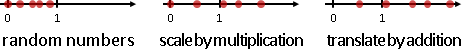```random(0, 2) + 3 = random(3, 5)
random(0, 2) - 3 = random(-3, -1)
random(0, 2) × 3 = random(0, 6)
random(0, 2) ÷ 3 = random(0, 2/3)
```

```base 10:
random[0, 4]
+ random[0, 2] × 10 = [0,4] or [10,14] or [20,24]
```
```base 2:
random[0, 2-1] × 2⁰
+ random[0, 2-1] × 2¹
+ random[0, 2-1] × 2² = random[0, 2³)
```
```prime base:
random[0, 2-1]
+ random[0, 3-1] × (2)
+ random[0, 5-1] × (2×3)
+ random[0, 7-1] × (2×3×5) = random[0, 2×3×5×7)
```

（後面章節介紹的Rejection Sampling。）

libstdc++的uniform_int_distribution，採用折衷方式，不是均勻分布。注意到註解的地方。

libc++的uniform_int_distribution，我沒有仔細看懂。

Random Tuple

2D Random Number```       regular：排列整齊。缺點是太過整齊，具有明顯紋路。
uniform：均勻分布。缺點是不夠整齊，偶有空白小格。
jittered：regular + uniform，每小格任取一點。
宏觀整齊適中，微觀仍不夠整齊，常常靠太近。
n-rook：n個城堡不互相攻擊。奇爛無比。
multi-jittered：每小格實施n-rook。稍微改善小處不夠整齊的問題。
Poisson disk：每一點到其他點的最短距離為定值d。整齊。慢。
best candidate：每一點到先前點的最短距離盡量長。不太整齊。快。
Hammersley：(i, i的二進位鏡射)。整齊適中。超快！
Halton：(i的a進位鏡射, i的b進位鏡射)，a與b互質。超快！
Sobol：我沒有研究。
```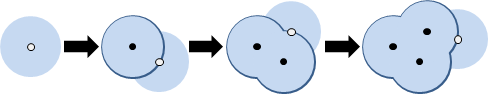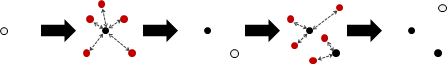(i, i的二進位鏡射)。

```i | i₍₂₎ |Φ(i)₍₂₎| Φ(i)
--|------|-------|------
1 |    1 | .1    | .5
2 |   10 | .01   | .25
3 |   11 | .11   | .75
4 |  100 | .001  | .125
5 |  101 | .101  | .635
6 |  110 | .011  | .325
7 |  111 | .111  | .875
8 | 1000 | .0001 | .0625

Φ(i): radical inverse of integer i
```

(i的a進位鏡射, i的b進位鏡射)，a與b互質。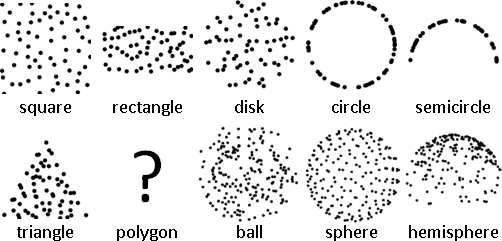Graphics3D[{PointSize[Large], Point[RandomPoint[Ball[], 200]]}, Boxed->False] Graphics3D[{PointSize[Large], Point[SpherePoints]}, Boxed->False] u1 := RandomReal[{0,1}]; u2 := RandomReal[{0,1}]; f[{u1_, u2_}] := {Sqrt[u1]*Cos[2*Pi*u2], Sqrt[u1]*Sin[2*Pi*u2], Sqrt[1-u1]}; pts = f /@ Table[{u1, u2}, {200}]; Graphics3D[{PointSize[Large], Point /@ pts}, Boxed->False]

Random Sequence

Random Sequence

Random Sampling

Random Sampling「隨機取樣」。創造大量隨機數字，符合指定分布。

UVa 12109